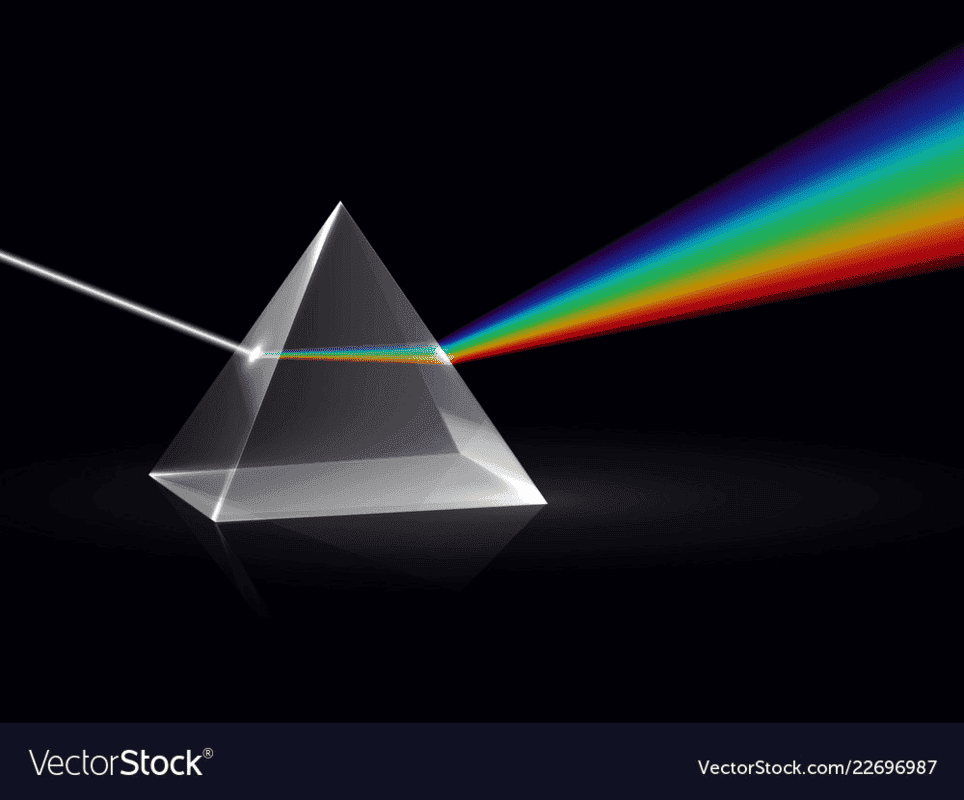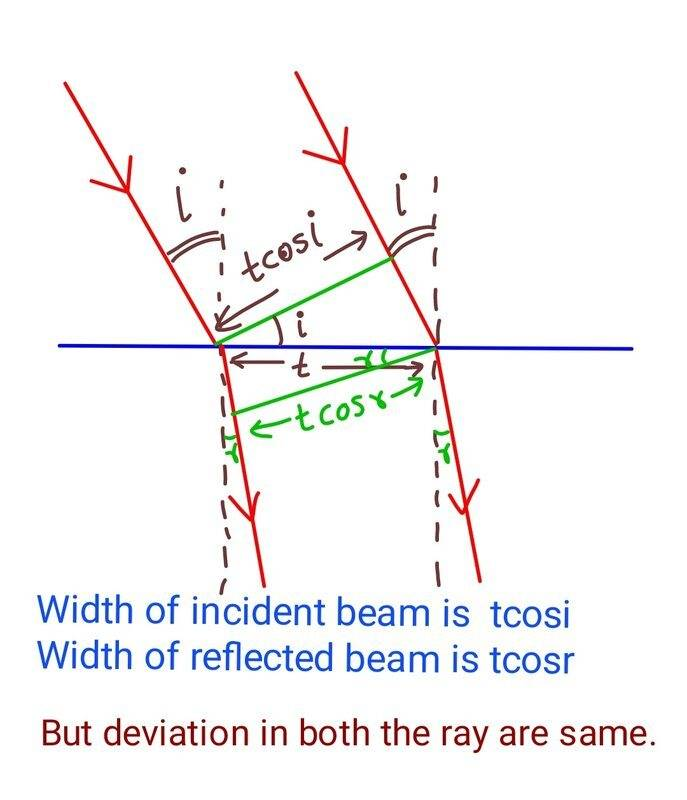# Size of a light beam before and after Refraction

• csirvi
In summary, the conversation discusses the size of a light beam before and after refraction from a medium. The question of whether the size remains the same or changes is raised, and the possibility of proving it through mathematical expression is also mentioned. Additionally, the topic of the angle of deviation and the width of the beam after refraction is brought up, with a request for a diagram or picture to further understand the situation.

#### csirvi

The size of light beam is same or different before and after refraction from a medium. If same then why we can not prove from mathematical expression. If not same why?

csirvi said:
Summary:: The size of light beam is same or different before and after refraction from a medium. If same then why we can not prove from mathematical expression. If not same why?

The size of light beam is same or different before and after refraction from a medium. If same then why we can not prove from mathematical expression. If not same why?
Doesn't look the same size to me...

https://cdn3.vectorstock.com/i/1000...rism-ray-rainbow-spectrum-vector-22696987.jpg•davenn and csirvi
Its helpful. . One more question is that if we take single color then the angle of deviation is same but also width of beam after refraction changes mathematically how that is physically possible ?

Can you post a picture or diagram of the situation you are asking about? Thanks.csirvi said:
then the angle of deviation is same but also width of beam after refraction changes mathematically
Does it? The width of the beam is the distance along a perpendicular to the direction of travel. Look carefully at the angles that you're taking teh cosine of... are these what you want?

Nugatory said:
Does it? The width of the beam is the distance along a perpendicular to the direction of travel. Look carefully at the angles that you're taking teh cosine of... are these what you want?
See width represent soze of beam yes that perpendicular to the Direction of travel.
My question is when the deviation angle same why the width is variable

berkeman said:
Can you post a picture or diagram of the situation you are asking about? Thanks.Posted the image.

berkeman said:
Can you post a picture or diagram of the situation you are asking about? Thanks.## 1. How does the size of a light beam change after refraction?

The size of a light beam can change after refraction, depending on the angle at which it enters the new medium. Generally, the light beam will become smaller when it enters a denser medium and larger when it enters a less dense medium. This is due to the change in the speed of the light as it passes through the different medium.

## 2. What causes the change in the size of a light beam after refraction?

The change in the size of a light beam after refraction is caused by the change in the speed of the light as it passes through a different medium. This change in speed is due to the change in the density of the medium, which affects the refractive index of the medium. The greater the change in refractive index, the larger the change in the size of the light beam.

## 3. Is there a formula to calculate the size of a light beam after refraction?

Yes, there is a formula to calculate the size of a light beam after refraction. It is known as the Snell's Law and it is given by n1sinθ1 = n2sinθ2, where n1 and n2 are the refractive indices of the two mediums, and θ1 and θ2 are the angles of incidence and refraction, respectively. This formula can be used to calculate the size of a light beam before and after refraction.

## 4. Can the size of a light beam change in the same medium?

No, the size of a light beam cannot change in the same medium. Refraction occurs when light passes through different mediums with different refractive indices. In the same medium, the refractive index remains constant, therefore the size of the light beam will also remain constant. However, the direction of the light beam can change due to diffraction or scattering.

## 5. How does the angle of incidence affect the size of a light beam after refraction?

The angle of incidence has a direct effect on the size of a light beam after refraction. The greater the angle of incidence, the greater the change in direction and the smaller the size of the light beam. This is because a larger angle of incidence means the light beam has to travel a longer distance in the new medium, resulting in more refraction and a smaller size of the light beam.